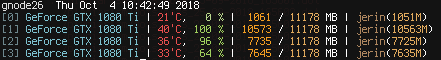I was stuck trying to load a checkpoint trained using DataParallel and a bunch of things seem to have worked so far for me. It took several iterations to fix, and I had to find the following after many attempts of searching. I feel there’s still some things I’m doing wrong, and am hoping this thread would help.

## DataParallel Training from start

I found that the usage(gpu0) = gpu1 + gpu2 + gpu3 + gpu0, which was due to computing loss outside. The following confused me, since it said loss can be outside.

The methods in the below thread meanwhile worked, once I put `model + loss` inside `DataParallel`, it balanced GPU memory to some extent.

## DataParallel Training from checkpoint

Everytime I tried to load it directly, I was getting out of memory error without even reaching the peak batch size I could achieve from the above setting. Eventually, loading weights to CPU first and then `load_state_dict` on the model from that worked to balance the memory imbalance across GPUs. I believe the following thread partially helped me out:

## Current Status

There is still an imbalance. I’m using 3 1080 Tis (1-3) with 11GB max memory. Attempts to increase further gives me OOM, while clearly  and  has free space.The following is the main training routine, at this point:

``````    def checkpoint(model, opt, checkpoint_path):
"model": model.module.state_dict(),
"opt": opt.state_dict()
}

with open(checkpoint_path, "wb+") as fp:

args = Args()
reduce = True
max_epochs = 80

model = LossGenerator(model, criterion)
checkpoint_path = "/scratch/jerin/best_checkpoint.pt"
model = model.to(device)
model = DataParallel(model)
if os.path.exists(checkpoint_path):

for epoch in tqdm(range(max_epochs), total=max_epochs, desc='epoch'):
meters["loss"].reset()
count = 0
for src, src_lens, tgt, tgt_lens in pbar:
count += 1
src, tgt = src.to(device), tgt.to(device)
loss = model(src, src_lens, tgt)
loss.sum().backward()
meters['loss'].update(loss.mean().item())
pbar.log(meters)
opt.step()

avg_loss = meters["loss"].avg
meters['epoch'].update(avg_loss)
checkpoint(model, opt, checkpoint_path)
``````

What are further improvements I could do? Could someone explain the internals of these things (loading, checkpoint) and best practices?

If I understand the source code of `DataParallel` correctly, you could try commenting this line `model = model.to(device)`. Also, try specifying the `output_device` for `DataParallel` to see if it works.

I tried a bunch of the combos.

• Commenting out `model = model.to(device)` gets me `Broadcast not implemented for CPUTensors...`
• Looking at the source now, if all outputs have to be gathered at one device, wouldn’t that increase the usage on device_ids anyway? Is there anyway to circumvent this?

Your main problem is you are using Adam. Adam parameters take memory from the main gpu to store its parameters. As far as I know there is nothing you can do. The bigger your model is, the worse this problem will be.
You can try to ask some developer if there is a way of spliting the memory usage of adam among all the gpus but, as you could see in the thread i opened, I got no answer.

1 Like

Can you link the thread corresponding to adam here? Or is it the one I mentioned above?